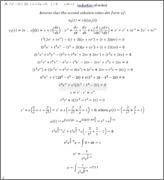# Reduction of Order Equation

## Homework Statement

t^2*y^'' -t(t+2)y^' + (t+2)y=0 , t>0; ysub1(t) = t

## Homework Equations

Reduction of order:

A second solution is assumed to be of the form:

ysub2(t) = v(t)*ysub1(t)

## The Attempt at a SolutionSo, the algebra in the first part of the process seemed to be correct, as it cancelled out all the "v" terms and left only derivatives of v. The problem seems to happen somewhere around when I turn it into a first order linear equation and attempt to multiply through by an integrating factor. As far as I, and a CAS are concerned, that integral at the end is not possible to take. This leaves the assumption that I completely goofed up my integrating factor. Where could I have gone wrong?

Mark44
Mentor

## Homework Statement

t^2*y^'' -t(t+2)y^' + (t+2)y=0 , t>0; ysub1(t) = t

## Homework Equations

Reduction of order:

A second solution is assumed to be of the form:

ysub2(t) = v(t)*ysub1(t)

## The Attempt at a SolutionSo, the algebra in the first part of the process seemed to be correct, as it cancelled out all the "v" terms and left only derivatives of v.
I don't think so. After substituting y2(t) = tv into the differential equation, and simplifying, I ended up with this equation:
##t^3v'' - t^3v' = 0##, which is pretty easy to solve.

I'm pretty confident that this is correct, as I went ahead to find ##y_2## and found that it satisfied the diff. eqn.

RyanTAsher said:
The problem seems to happen somewhere around when I turn it into a first order linear equation and attempt to multiply through by an integrating factor. As far as I, and a CAS are concerned, that integral at the end is not possible to take. This leaves the assumption that I completely goofed up my integrating factor. Where could I have gone wrong?

Okay, I re-did all my algebra and I made the mistake somewhere distributing in the middle term. Thank you for your help.